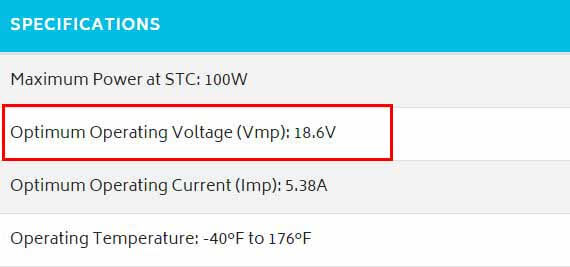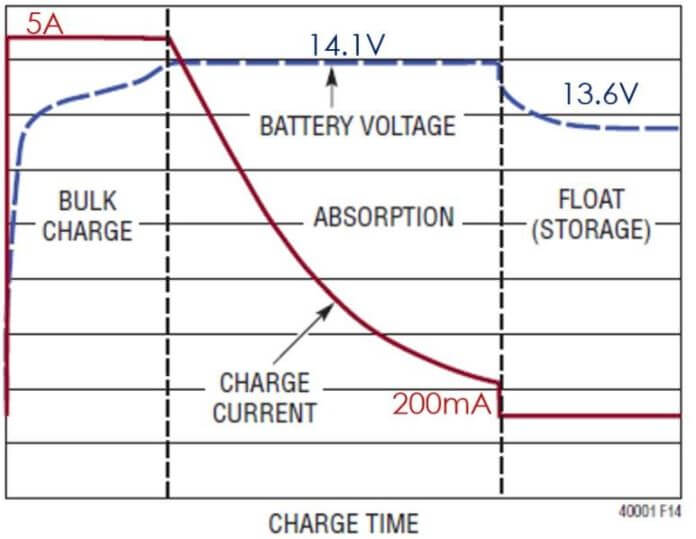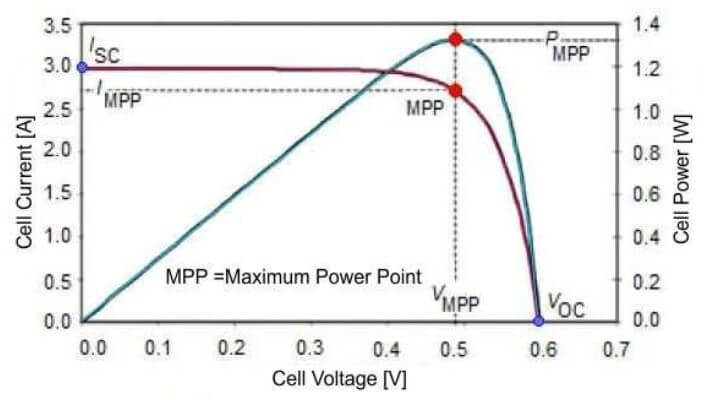# How does a solar charge controller work and why do you need one?

A solar charge controller also called a regulator, is an electronic device used in solar energy systems to protect the battery.

Solar charge controllers are mainly used to keep batteries from overcharging and over-discharging. However, newer MPPT charge controllers can also decrease power production losses.

In this article, I’ll go over the reasons you need a solar charge controller and the types of charge controllers on the market.

## What does a solar charge controller do and why do you need it?

Solar charge controllers connect solar panels to the batteries to protect the batteries from overcharging and over-discharging. Charge controllers also protect solar panels at night when they stop producing electricity.

Let’s see what this means.

### Preventing battery overcharging:

A 12V solar panel is used to charge a 12V battery, the problem is that the 12V is “nominal”. This means that 12V is not actually the real voltage of the solar panel, but rather the voltage used to categorize the panel.

Provided that there’s sunlight, when connected to a load, the actual voltage produced by a 12V solar panel is somewhere between 16 and 19 Volts.Specification sheet of a 100W-12V solar panel from Renogy.

While the charging voltage that the battery requires does not exceed 14.4V.12V Lead Acid battery charging voltage and current

The voltage of a 12V solar panel is intended by the manufacturers to always be higher than that of a 12V battery. However, this in and of itself creates a problem.

Since a fully charged 12V battery has a voltage of about 13V at its terminals, a directly connected 12V solar panel will try and further push that voltage up to about 18V.

This difference in voltage will force an excessive amount of power into the battery, causing it to overheat and irreversibly damage it.

Overheating a battery will, at the very least, shorten its cycle life. Unregulated charging of a battery can potentially result in something like this:Battery damaged from overcharging

This can be easily prevented with a solar charge controller connected between the solar panels and battery. The charge controller takes the output voltage from the PV array and regulates it so that it matches the voltage of the battery. This ensures that the battery is not overcharging.

### Preventing battery over-discharging:

Without a good battery protection circuit, a battery can actually keep discharging into a load even after being completely depleted.

Battery life and its depth of discharge (DoD) are pretty much correlated. Every time you discharge a battery more than its full capacity or even more than the recommended depth of discharge, it will lose a percentage of its capacity and life.

Thankfully, solar charge controllers have an independent DC load input that can be disconnected and reconnected if needed.Solar charge controllers can prevent battery over-discharging by disconnecting the DC loads when the battery is at a low capacity. This is mainly done through the Low Voltage Disconnect (LVD) feature.

The lower the state of charge (SoC) of a battery, the lower its voltage. In the image below, you can see the voltages of a typical Lead-Acid battery vs its state of charge:Solar charge controllers with the low voltage disconnect feature typically have a default LVD voltage of around 11V. Meaning once the battery’s voltage is at 11V, the load is automatically disconnected. But in most cases, the load disconnect voltage can be adjusted to meet your preferences.

### Preventing batteries from discharging into solar panels:

At night when there is no light whatsoever, the voltage from a solar panel becomes negligible. Since current tends to flow from higher voltages to lower voltages, if a charged battery is directly connected to the solar panel, the latter will act as a load and start drawing power from the battery.

If this occurs, 2 things can happen:

• The battery can potentially be depleted overnight and can be at risk of over-discharge.
• Solar panels can potentially be damaged.

In the earlier days – to prevent this from happening – solar system owners would install a blocking diode in series between solar panels and battery bank.

However, today’s solar charge controllers have a blocking diode embedded in their electronic circuitry. So you will not have to worry about installing a blocking diode if you have a charge controller.

## What are the types of solar charge controllers?

In today’s market, there are two types of solar charge controller technologies:

Both of these solar charge controllers come in different sizes and can handle different size solar systems.

And both of these solar charge controllers will do the job, but the way each of them operates can make a big difference in power production.

### What is a PWM charge controller and how does it work?

A PWM solar charge controller is a smart ON/OFF switch that regulates the DC voltage from the solar panels to match that of the battery.When your battery is almost charged, a PWM controller lowers the voltage from the solar panels by switching ON and OFF (i.e. connecting and disconnecting the solar panels). This lowers the average DC output voltage and consequently prevents overcharging.Since the voltage of a battery is relative to its state of charge, PWM chargers measure the state of charge of the battery by sensing its voltage.

When the voltage of the battery is low (10-13V), the PWM charge controller increases the duty cycle (time period for which the panels are connected to the battery). When the voltage is high (13.6V-14V), the PWM decreases the duty cycle until the desirable voltage is maintained.

In this process, the PWM charge controller ensures the safe charging of the battery, but there’s an issue. Since power is the product of voltage and current, if you decrease the voltage by a certain ratio, the power will decrease by that same ratio.

This results in considerable power losses which with big systems can be very costly.

Although they cost more, MPPT charge controllers fix this problem.

### What is an MPPT charge controller and how does it work?

MPPT solar charge controllers are DC-DC converters that track the voltage and current for which the output power from the solar panels is maximal.

MPPT chargers maximize the output power from the solar array, then – without changing that amount of power – transforms it from high voltage power to lower voltage power.

In other words, an MPPT charge controller lowers the voltage from the solar array to match the voltage of the battery bank, while also raising the current.

This ensures the safe charging of the battery bank and negligible power losses.

So, essentially an MPPT charge controller has 2 circuits:

#### Maximum Power Point Tracking:

The image below shows the IV and PV characteristics of a solar cell:If you change the output voltage from a PV cell, its output current also changes. And since power is the product of voltage and current, there is a certain operating point for which you get maximum power. That point is called the Maximum Power Point (MPP).

MPPTs constantly track this point by varying the voltage of the PV cell, once that power point is found, the voltage is maintained. By maintaining that voltage, the MPPT maintains maximum PV output power.

#### DC to DC conversion:

Aside from the maximum power point tracking, another advantage that an MPPT controller has over a PWM controller is that while it lowers the voltage it also raises the current by the same ratio.

This protects the battery and prevents any power losses at the same time

MPPTs do this by:

1. Converting the initial DC input to AC.
2. Running the AC voltage through a step-down transformer, which decreases the voltage and increases the current by the same ratio.
3. And finally converting the AC voltage back to a safer DC voltage.

The output of this DC to DC conversion is the same power generated by the solar panels with virtually no losses.

The disadvantage however is that all of this superior technology comes at a price.

With MPPT charge controllers, you’ll have to pay at least 5 to 6 times more than what you would pay for a PWM charge controller.

## How much does a solar charge controller cost?

The price of a solar charge controller depends on the size of your system and the type of controller you’re looking for. A PWM charge controller can cost anywhere from \$15 to \$100, while MPPT controllers cost anywhere from \$100 to \$700.

If you know which type of charge controller you’re looking for, The price depends mainly on the size of your system and how big of a charge controller you need.

To put things into perspective, let’s consider the following solar system as an example:

2 100W-12V solar panels are wired in parallel and connected to a 12V battery.

• Solar array Wattage: 200W
• Solar array Voltage: 12V
• Solar array Short-Circuit Current: 11.72A
• Battery Voltage: 12V

Under standard test conditions, A system with these specifications would roughly require a 15A solar charge controller.

If we were to use a PWM for this system, a good choice would be to use this 20A PWM charge controller which only costs \$17.

If we wanted to use an MPPT, this \$93 20A MPPT charge controller from Renogy would be a good fit.

So before you really know how much it’ll cost you to buy a charge controller, you’ll have to size it first.

In case you don’t know how to size a charge controller, the section below briefly explains how to size both PWMs and MPPTs with links to more detailed guides.

## What size charge controller do I need?

The size of the solar charge controller depends mainly on how big of a system you have. However, since PWM and MPPT charge controllers are 2 different technologies, each of them is sized in a different way.

### How to size a PWM solar charge controller:

To size a PWM charge controller, you have to calculate the maximum amount of current your solar array is expected to produce. The amperage of the PWM charger you choose has to be equal to or higher than that maximum current.

This maximum current can be calculated as follows:

Maximum Current = (Solar Array Short-Circuit Current) x 1.25

• Solar Array Short-Circuit Current: This can be calculated by multiplying the Short-Circuit Current specified on your solar panels by the number of parallel strings in your solar array.
• 1.25 is NEC’s safety factor.

The page includes an example and a calculator that will display a direct Amazon link to the PWM controller you need.

### How to size an MPPT solar charge controller:

MPPT charge controllers transform the power from your solar panels to a lower voltage/higher current power; to size an MPPT controller, you have to divide the wattage of your solar panels by the charging voltage of your battery bank.

MPPT Amperage Rating = (Solar Array Wattage) ÷ (Battery Bank charging voltage)

• Solar Array Wattage: This is the power rating of all of your solar panels put together.
• Battery Bank Charging Voltage: This is the voltage at the terminals of your battery bank when it’s charging (around 14.4V for a 12V battery bank).

MPPT charge controllers are also limited by a maximum input voltage. So when sizing an MPPT, you also have to calculate the maximum voltage from your solar panels. This can be done by multiplying the Open-Circuit voltage of your solar array by a temperature correction factor.